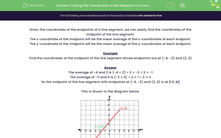# Find the Coordinates of the Midpoint of a Line Segment

In this worksheet, students will find the coordinates of the midpoint of a line segment, given the coordinates of its endpoints.Key stage:  KS 3

Curriculum topic:   Algebra

Curriculum subtopic:   Use Coordinates in All Four Quadrants

Popular topics:   Algebra worksheets

Difficulty level:#### Worksheet Overview

Given the coordinates of the endpoints of a line segment, we can easily find the coordinates of the midpoint of the line segment.

The x-coordinate of the midpoint will be the mean average of the x-coordinates of each endpoint.

The y-coordinate of the midpoint will be the mean average of the y-coordinates of each endpoint.

Example

Find the coordinates of the midpoint of the line segment whose endpoints are at (-4, -3) and (2, 4)

The average of -4 and 2 is (-4 + 2) ÷ 2 = -2 ÷ 2 = -1

The average of -3 and 4 is (-3 + 4) ÷ 2 = 1 ÷ 2 = 0.5

So the midpoint of the line segment with endpoints at (-4, -3) and (2, 4) is at (-1, 0.5)

This is shown in the diagram below.The most tricky part of it is making sure that you don't get muddled with your negative numbers.

Want a bit more help with this before you begin? Why not watch this short video?

### What is EdPlace?

We're your National Curriculum aligned online education content provider helping each child succeed in English, maths and science from year 1 to GCSE. With an EdPlace account you’ll be able to track and measure progress, helping each child achieve their best. We build confidence and attainment by personalising each child’s learning at a level that suits them.

Get started